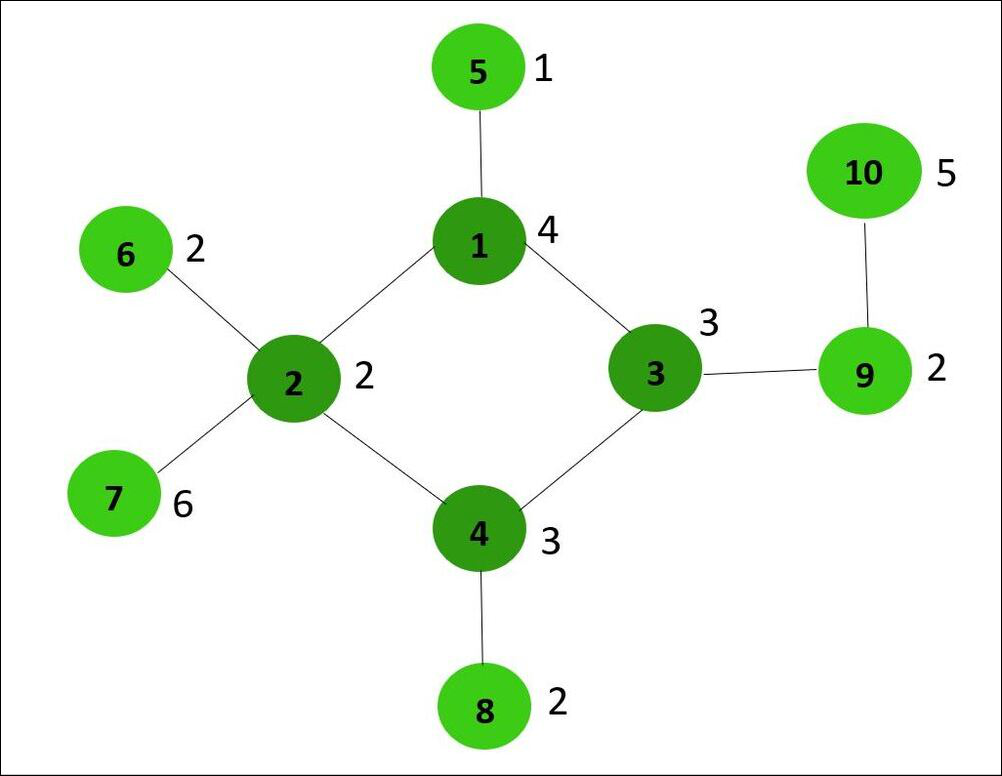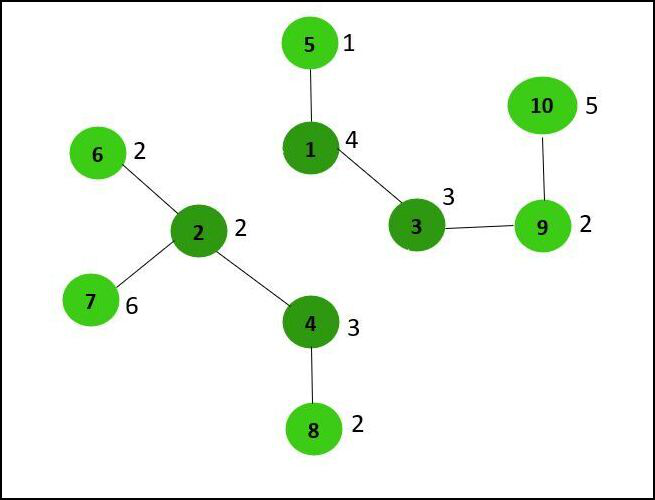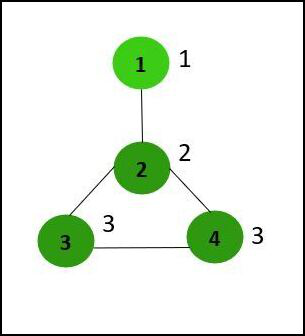# Check if equal sum components can be obtained from given Graph by removing edges from a Cycle

• Difficulty Level : Hard
• Last Updated : 24 Jun, 2021

Given an undirected graph with N vertices and N edges that contain only one cycle, and an array arr[] of size N, where arr[i] denotes the value of the ith node, the task is to check if the cycle can be divided into two components such that the sum of all the node values in both the components is the same.

Examples:

Input: N = 10, arr[] = {4, 2, 3, 3, 1, 2, 6, 2, 2, 5}, edges[] = {{1, 2}, {1, 5}, {1, 3}, {2, 6}, {2, 7}, {2, 4}, {4, 8}, {4, 3}, {3, 9}, {9, 10} }Output: Yes
Explanation: By removing the edges 1-2 and 3-4, the sum of all the node values of both the generated components is equal to 15.Input: N= 4, arr[] = {1, 2, 3, 3}, edges[] = {{1, 2}, {2, 3}, {3, 4}, {2, 4}}Output: No
Explanation: No possible way exists to obtain two equal sum components by removing an edges from the cycle.

Approach: The idea to solve this problem is to first find the nodes which are part of the cycle. Then, add the value of each node that is not a part of the cycle to its nearest node in the cycle. The final step involves checking whether the cycle can be divided into two equal sum components. Below are the steps:

• The first step is to find all the nodes which are a part of a cycle using detect cycle in an undirected graph using DFS.
• Perform the DFS Traversal on the given graph and do the following:
• Mark the current node as visited. For each unvisited node connected to the current node recursively perform the DFS Traversal for each node.
• If the adjacent node of the current node is already visited and is not the same as the previous node of the current node then it means that the current node is part of the cycle. Backtrack till this specific adjacent node is reached to find all nodes that are part of the cycle and store them in a vector inCycle[].
• Find the sum of values for each node in the inCycle[] and add this sum to the current inCycle[] node value.
• Find the total sum of values of all the nodes present in inCycle[] and store it in a variable totalSum. If the value of totalSum is odd then print “No” because the cycle with an odd sum cannot be divided into two equal sum components.
• Otherwise, check if the value of totalSum/2 is present in inCycle[] then print “Yes” Else print “No”.

Below is the implementation of the above approach:

## C++

 `// C++ program for the above approach``#include ``using` `namespace` `std;` `// Recursive function to find all the``// nodes that are part of the cycle``void` `findNodesinCycle(``int` `u, ``bool``* vis,``                      ``int``* prev,``                      ``vector<``int``> adj[],``                      ``vector<``int``>& inCycle)``{``    ``// Mark current node as visited``    ``vis[u] = ``true``;` `    ``for` `(``int` `v : adj[u]) {` `        ``// If node v is not visited``        ``if` `(!vis[v]) {` `            ``prev[v] = u;` `            ``// Recursively find cycle``            ``findNodesinCycle(v, vis, prev,``                             ``adj, inCycle);` `            ``// If cycle is detected then``            ``// return the previous node``            ``if` `(!inCycle.empty())``                ``return``;``        ``}` `        ``// If node is already visited``        ``// and not equal to prev[u]``        ``else` `if` `(v != prev[u]) {``            ``int` `curr = u;``            ``inCycle.push_back(curr);` `            ``// Backtrack all vertices``            ``// that are part of cycle``            ``// and store them in inCycle``            ``while` `(curr != v) {``                ``curr = prev[curr];``                ``inCycle.push_back(curr);``            ``}` `            ``// As the cycle is detected``            ``// return the previous node``            ``return``;``        ``}``    ``}``}` `// Function to add the value of each``// node which is not part of the cycle``// to its nearest node in the cycle``int` `sumOfnonCycleNodes(``    ``int` `u, vector<``int``> adj[],``    ``vector<``int``> inCycle, ``bool``* vis,``    ``int` `arr[])``{``    ``// Mark the current node as visited``    ``vis[u] = ``true``;` `    ``// Stores the value of required sum``    ``int` `sum = 0;` `    ``for` `(``int` `v : adj[u]) {` `        ``// If v is not already visited``        ``// and not present in inCycle``        ``if` `(!vis[v]` `            ``&& find(inCycle.begin(),``                    ``inCycle.end(), v)``                   ``== inCycle.end()) {` `            ``// Add to sum and call the``            ``// function recursively``            ``sum += (arr[v - 1]``                    ``+ sumOfnonCycleNodes(``                          ``v, adj, inCycle,``                          ``vis, arr));``        ``}``    ``}` `    ``// Return the value of sum``    ``return` `sum;``}` `// Function that add the edges``// to the graph``void` `addEdge(vector<``int``> adj[],``             ``int` `u, ``int` `v)``{``    ``adj[u].push_back(v);``    ``adj[v].push_back(u);``}` `// Utility Function to check if the cycle``// can be divided into two same sum``bool` `isBreakingPossible(vector<``int``> adj[],``                        ``int` `arr[], ``int` `N)``{``    ``// Stores all the nodes that are``    ``// part of the cycle``    ``vector<``int``> inCycle;` `    ``// Array to check if a node is``    ``// already visited or not``    ``bool` `vis[N + 1];` `    ``// Initialize vis to false``    ``memset``(vis, ``false``, ``sizeof` `vis);` `    ``// Array to store the previous node``    ``// of the current node``    ``int` `prev[N + 1];` `    ``// Initialize prev to 0``    ``memset``(prev, 0, ``sizeof` `prev);` `    ``// Recursive function call``    ``findNodesinCycle(1, vis, prev,``                     ``adj, inCycle);` `    ``memset``(vis, ``false``, ``sizeof` `vis);` `    ``// Update value of each inCycle``    ``// node``    ``for` `(``int` `u : inCycle) {` `        ``// Add sum of values of all``        ``// required node to current``        ``// inCycle node value``        ``arr[u - 1] += sumOfnonCycleNodes(``            ``u, adj, inCycle, vis, arr);``    ``}` `    ``// Stores total sum of values of``    ``// all nodes present in inCycle``    ``int` `tot_sum = 0;` `    ``// Find the total required sum``    ``for` `(``int` `node : inCycle) {``        ``tot_sum += arr[node - 1];``    ``}` `    ``// If value of tot_sum is odd``    ``// then return false``    ``if` `(tot_sum % 2 != 0)``        ``return` `false``;` `    ``int` `req_sum = tot_sum / 2;` `    ``// Create an empty map``    ``unordered_map<``int``, ``int``> map;` `    ``// Initialise map``    ``map = -1;` `    ``// Maintain the sum of values of``    ``// nodes so far``    ``int` `curr_sum = 0;` `    ``for` `(``int` `i = 0; i < inCycle.size(); i++) {` `        ``// Add the current node value``        ``// to curr_sum``        ``curr_sum += arr[inCycle[i] - 1];` `        ``// If curr_sum - req_sum in map``        ``// then there is a subarray of``        ``// nodes with sum of their values``        ``// equal to req_sum``        ``if` `(map.find(curr_sum - req_sum)``            ``!= map.end()) {``            ``return` `true``;``        ``}` `        ``map[curr_sum] = i;``    ``}` `    ``// If no such subarray exists``    ``return` `false``;``}` `// Function to check if the cycle can``// be divided into two same sum``void` `checkCycleDivided(``int` `edges[],``                       ``int` `arr[],``                       ``int` `N)``{``    ``vector<``int``> adj[N + 1];` `    ``// Traverse the given edges``    ``for` `(``int` `i = 0; i < N; i++) {` `        ``int` `u = edges[i];``        ``int` `v = edges[i];` `        ``// Add the edges``        ``addEdge(adj, u, v);``    ``}` `    ``// Print the result``    ``cout << (isBreakingPossible(``                 ``adj, arr, N)``                 ``? ``"Yes"``                 ``: ``"No"``);``}` `// Driver Code``int` `main()``{``    ``int` `N = 10;``    ``int` `edges[] = { { 1, 2 }, { 1, 5 }, { 1, 3 }, { 2, 6 }, { 2, 7 }, { 2, 4 }, { 4, 8 }, { 4, 3 }, { 3, 9 }, { 9, 10 } };``    ``int` `arr[] = { 4, 2, 3, 3, 1,``                  ``2, 6, 2, 2, 5 };` `    ``// Function Call``    ``checkCycleDivided(edges, arr, N);` `    ``return` `0;``}`

## Java

 `// Java program for the above approach``import` `java.util.*;``class` `GFG``{` `  ``// Recursive function to find all the``  ``// nodes that are part of the cycle``  ``static` `void` `findNodesinCycle(``int` `u, ``boolean``[] vis,``                               ``int``[] prev,``                               ``ArrayList> adj,``                               ``ArrayList inCycle)``  ``{``    ` `    ``// Mark current node as visited``    ``vis[u] = ``true``;``    ``for` `(``int` `v : adj.get(u))``    ``{` `      ``// If node v is not visited``      ``if` `(!vis[v])``      ``{``        ``prev[v] = u;` `        ``// Recursively find cycle``        ``findNodesinCycle(v, vis, prev,``                         ``adj, inCycle);` `        ``// If cycle is detected then``        ``// return the previous node``        ``if` `(inCycle.size() > ``0``)``          ``return``;``      ``}` `      ``// If node is already visited``      ``// and not equal to prev[u]``      ``else` `if` `(v != prev[u])``      ``{``        ``int` `curr = u;``        ``inCycle.add(curr);` `        ``// Backtrack all vertices``        ``// that are part of cycle``        ``// and store them in inCycle``        ``while` `(curr != v)``        ``{``          ``curr = prev[curr];``          ``inCycle.add(curr);``        ``}` `        ``// As the cycle is detected``        ``// return the previous node``        ``return``;``      ``}``    ``}``  ``}` `  ``// Function to add the value of each``  ``// node which is not part of the cycle``  ``// to its nearest node in the cycle``  ``static` `int` `sumOfnonCycleNodes(``    ``int` `u, ArrayList> adj,``    ``ArrayList inCycle, ``boolean``[] vis,``    ``int` `arr[])``  ``{` `    ``// Mark the current node as visited``    ``vis[u] = ``true``;` `    ``// Stores the value of required sum``    ``int` `sum = ``0``;` `    ``for` `(``int` `v : adj.get(u))``    ``{` `      ``// If v is not already visited``      ``// and not present in inCycle``      ``if` `(!vis[v]``          ``&& !inCycle.contains(v))``      ``{` `        ``// Add to sum and call the``        ``// function recursively``        ``sum += (arr[v - ``1``] + sumOfnonCycleNodes(``          ``v, adj, inCycle, vis, arr));``      ``}``    ``}` `    ``// Return the value of sum``    ``return` `sum;``  ``}` `  ``// Utility Function to check if the cycle``  ``// can be divided into two same sum``  ``static` `boolean` `isBreakingPossible(ArrayList> adj,``                                    ``int` `arr[], ``int` `N)``  ``{` `    ``// Stores all the nodes that are``    ``// part of the cycle``    ``ArrayList inCycle = ``new` `ArrayList<>();` `    ``// Array to check if a node is``    ``// already visited or not``    ``boolean``[] vis = ``new` `boolean``[N + ``1``];` `    ``// Array to store the previous node``    ``// of the current node``    ``int``[] prev = ``new` `int``[N + ``1``];` `    ``// Recursive function call``    ``findNodesinCycle(``1``, vis, prev,``                     ``adj, inCycle);` `    ``Arrays.fill(vis,``false``);` `    ``// Update value of each inCycle``    ``// node``    ``for` `(Integer u : inCycle)``    ``{` `      ``// Add sum of values of all``      ``// required node to current``      ``// inCycle node value``      ``arr[u - ``1``] += sumOfnonCycleNodes(``        ``u, adj, inCycle, vis, arr);``    ``}` `    ``// Stores total sum of values of``    ``// all nodes present in inCycle``    ``int` `tot_sum = ``0``;` `    ``// Find the total required sum``    ``for` `(``int` `node : inCycle)``    ``{``      ``tot_sum += arr[node - ``1``];``    ``}` `    ``// If value of tot_sum is odd``    ``// then return false``    ``if` `(tot_sum % ``2` `!= ``0``)``      ``return` `false``;``    ``int` `req_sum = tot_sum / ``2``;` `    ``// Create an empty map``    ``Map map = ``new` `HashMap<>();` `    ``// Initialise map``    ``map.put(``0``, -``1``);` `    ``// Maintain the sum of values of``    ``// nodes so far``    ``int` `curr_sum = ``0``;``    ``for` `(``int` `i = ``0``; i < inCycle.size(); i++)``    ``{` `      ``// Add the current node value``      ``// to curr_sum``      ``curr_sum += arr[inCycle.get(i) - ``1``];` `      ``// If curr_sum - req_sum in map``      ``// then there is a subarray of``      ``// nodes with sum of their values``      ``// equal to req_sum``      ``if` `(map.containsKey(curr_sum - req_sum))``      ``{``        ``return` `true``;``      ``}``      ``map.put(curr_sum, i);``    ``}` `    ``// If no such subarray exists``    ``return` `false``;``  ``}` `  ``// Function to check if the cycle can``  ``// be divided into two same sum``  ``static` `void` `checkCycleDivided(``int` `edges[][],``                                ``int` `arr[], ``int` `N)``  ``{``    ``ArrayList> adj = ``new` `ArrayList<>();` `    ``for``(``int` `i = ``0``; i <= N; i++)``      ``adj.add(``new` `ArrayList<>());` `    ``// Traverse the given edges``    ``for` `(``int` `i = ``0``; i < N; i++)``    ``{` `      ``int` `u = edges[i][``0``];``      ``int` `v = edges[i][``1``];` `      ``// Add the edges``      ``adj.get(u).add(v);``      ``adj.get(v).add(u);``    ``}` `    ``// Print the result``    ``System.out.print(isBreakingPossible(``      ``adj, arr, N) ? ``"Yes"` `: ``"No"``);``  ``}` `  ``// Driver code``  ``public` `static` `void` `main (String[] args)``  ``{``    ``int` `N = ``10``;``    ``int` `edges[][] = { { ``1``, ``2` `}, { ``1``, ``5` `},``                     ``{ ``1``, ``3` `}, { ``2``, ``6` `},``                     ``{ ``2``, ``7` `}, { ``2``, ``4` `},``                     ``{ ``4``, ``8` `}, { ``4``, ``3` `},``                     ``{ ``3``, ``9` `}, { ``9``, ``10` `} };``    ``int` `arr[] = { ``4``, ``2``, ``3``, ``3``, ``1``,``                 ``2``, ``6``, ``2``, ``2``, ``5` `};` `    ``// Function Call``    ``checkCycleDivided(edges, arr, N);``  ``}``}` `// This code is contributed by offbeat`

## Python3

 `# Python3 program for the above approach` `# Recursive function to find all the``# nodes that are part of the cycle``def` `findNodesinCycle(u):``    ``global` `adj, pre, inCycle, vis``    ``vis[u] ``=` `True``    ``for` `v ``in` `adj[u]:` `        ``# If node v is not visited``        ``if` `(``not` `vis[v]):``            ``pre[v] ``=` `u` `            ``# Recursively find cycle``            ``findNodesinCycle(v)` `            ``# If cycle is detected then``            ``# return the previous node``            ``if` `(``len``(inCycle) > ``0``):``                ``return` `        ``# If node is already visited``        ``# and not equal to prev[u]``        ``elif` `(v !``=` `pre[u]):``            ``curr ``=` `u``            ``inCycle.append(curr)` `            ``# Backtrack all vertices``            ``# that are part of cycle``            ``# and store them in inCycle``            ``while` `(curr !``=` `v):``                ``curr ``=` `pre[curr]``                ``inCycle.append(curr)` `            ``# As the cycle is detected``            ``# return the previous node``            ``return` `# Function to add the value of each``# node which is not part of the cycle``# to its nearest node in the cycle``def` `sumOfnonCycleNodes(u, arr):``    ``global` `adj, pre, inCycle, vis``    ``vis[u] ``=` `True` `    ``# Stores the value of required sum``    ``sum` `=` `0``    ``for` `v ``in` `adj[u]:` `        ``# If v is not already visited``        ``# and not present in inCycle``        ``if` `(``not` `vis[v]) ``and` `(v ``not` `in` `inCycle):` `            ``# Add to sum and call the``            ``# function recursively``            ``sum` `+``=` `(arr[v ``-` `1``] ``+` `sumOfnonCycleNodes(v, arr))` `    ``# Return the value of sum``    ``return` `sum` `# Function that add the edges``# to the graph``def` `addEdge(u, v):``    ``global` `adj``    ``adj[u].append(v)``    ``adj[v].append(u)` `# Utility Function to check if the cycle``# can be divided into two same sum``def` `isBreakingPossible(arr, N):``    ` `    ``# Stores all the nodes that are``    ``global` `adj, vis, pre` `    ``# Recursive function call``    ``findNodesinCycle(``1``,)` `    ``for` `i ``in` `range``(N ``+` `1``):``        ``vis[i] ``=` `False` `    ``# Update value of each inCycle``    ``# node``    ``for` `u ``in` `inCycle:` `        ``# Add sum of values of all``        ``# required node to current``        ``# inCycle node value``        ``arr[u ``-` `1``] ``+``=` `sumOfnonCycleNodes(u, arr)` `    ``# Stores total sum of values of``    ``# all nodes present in inCycle``    ``tot_sum ``=` `0` `    ``# Find the total required sum``    ``for` `node ``in` `inCycle:``        ``tot_sum ``+``=` `arr[node ``-` `1``]` `    ``# If value of tot_sum is odd``    ``# then return false``    ``if` `(tot_sum ``%` `2` `!``=` `0``):``        ``return` `False` `    ``req_sum ``=` `tot_sum ``/``/` `2` `    ``# Create an empty map``    ``map` `=` `{}` `    ``# Initialise map``    ``map``[``0``] ``=` `-``1` `    ``# Maintain the sum of values of``    ``# nodes so far``    ``curr_sum ``=` `0``    ``for` `i ``in` `range``(``len``(inCycle)):` `        ``# Add the current node value``        ``# to curr_sum``        ``curr_sum ``+``=` `arr[inCycle[i] ``-` `1``]` `        ``# If curr_sum - req_sum in map``        ``# then there is a subarray of``        ``# nodes with sum of their values``        ``# equal to req_sum``        ``if` `((curr_sum ``-` `req_sum) ``in` `map``):``            ``return` `True``        ``map``[curr_sum] ``=` `i` `    ``# If no such subarray exists``    ``return` `False` `# Function to check if the cycle can``# be divided into two same sum``def` `checkCycleDivided(edges, arr, N):``    ``global` `adj` `    ``# Traverse the given edges``    ``for` `i ``in` `range``(N):` `        ``u ``=` `edges[i][``0``]``        ``v ``=` `edges[i][``1``]` `        ``# Add the edges``        ``addEdge(u, v)` `    ``# Print the result``    ``print``(``"Yes"` `if` `isBreakingPossible(arr, N) ``else` `"No"``)` `# Driver Code``if` `__name__ ``=``=` `'__main__'``:``    ``N ``=` `10``    ``edges``=` `[[``1``, ``2``], [``1``, ``5``], [``1``, ``3``],``            ``[``2``, ``6``], [``2``, ``7``], [``2``, ``4``],``            ``[``4``, ``8``], [``4``, ``3``], [``3``, ``9``],``            ``[``9``, ``10``]]``    ``arr, adj, vis ``=`  `[``4``, ``2``, ``3``, ``3``, ``1``,``                     ``2``, ``6``, ``2``, ``2``, ``5``], [[] ``for` `i ``in` `range``(N ``+` `1``)], [``False` `for` `i ``in` `range``(N``+``1``)]` `    ``inCycle, pre ``=``[], [``0` `for` `i ``in` `range``(N``+``1``)]``    ``checkCycleDivided(edges, arr, N)` `    ``# This code is contributed by mohit kumar 29`

## C#

 `// C# program for the above approach``using` `System;``using` `System.Collections.Generic;` `public` `class` `GFG``{` `  ``// Recursive function to find all the``  ``// nodes that are part of the cycle``  ``static` `void` `findNodesinCycle(``int` `u, ``bool``[] vis,``int``[] prev,``                               ``List> adj,List<``int``> inCycle)``  ``{` `    ``// Mark current node as visited``    ``vis[u] = ``true``;` `    ``foreach``(``int` `v ``in` `adj[u])``    ``{` `      ``// If node v is not visited``      ``if` `(!vis[v])``      ``{``        ``prev[v] = u;` `        ``// Recursively find cycle``        ``findNodesinCycle(v, vis, prev,adj, inCycle);` `        ``// If cycle is detected then``        ``// return the previous node``        ``if` `(inCycle.Count > 0)``          ``return``;``      ``}` `      ``// If node is already visited``      ``// and not equal to prev[u]``      ``else` `if` `(v != prev[u])``      ``{``        ``int` `curr = u;``        ``inCycle.Add(curr);` `        ``// Backtrack all vertices``        ``// that are part of cycle``        ``// and store them in inCycle` `        ``while` `(curr != v)``        ``{``          ``curr = prev[curr];``          ``inCycle.Add(curr);``        ``}` `        ``// As the cycle is detected``        ``// return the previous node``        ``return``;``      ``}``    ``}``  ``}` `  ``// Function to add the value of each``  ``// node which is not part of the cycle``  ``// to its nearest node in the cycle``  ``static` `int` `sumOfnonCycleNodes(``int` `u, List> adj,List<``int``> inCycle, ``bool``[] vis,``int``[] arr)``  ``{``    ``// Mark the current node as visited``    ``vis[u] = ``true``;` `    ``// Stores the value of required sum``    ``int` `sum = 0;` `    ``foreach``(``int` `v ``in` `adj[u])``    ``{``      ``// If v is not already visited``      ``// and not present in inCycle``      ``if` `(!vis[v] && !inCycle.Contains(v))``      ``{``        ``// Add to sum and call the``        ``// function recursively``        ``sum += (arr[v - 1] + sumOfnonCycleNodes(v, adj, inCycle, vis, arr));``      ``}``    ``}` `    ``// Return the value of sum``    ``return` `sum;``  ``}` `  ``// Utility Function to check if the cycle``  ``// can be divided into two same sum``  ``static` `bool` `isBreakingPossible(List> adj,``int``[] arr, ``int` `N)``  ``{` `    ``// Stores all the nodes that are``    ``// part of the cycle``    ``List<``int``> inCycle = ``new` `List<``int``>();` `    ``// Array to check if a node is``    ``// already visited or not``    ``bool``[] vis = ``new` `bool``[N + 1];` `    ``// Array to store the previous node``    ``// of the current node``    ``int``[] prev = ``new` `int``[N + 1];` `    ``// Recursive function call``    ``findNodesinCycle(1, vis, prev, adj, inCycle);` `    ``// Update value of each inCycle``    ``// node``    ``foreach``(``int` `u ``in` `inCycle)``    ``{` `      ``// Add sum of values of all``      ``// required node to current``      ``// inCycle node value``      ``arr[u - 1] += sumOfnonCycleNodes(u, adj, inCycle, vis, arr);``    ``}` `    ``// Stores total sum of values of``    ``// all nodes present in inCycle``    ``int` `tot_sum = 0;` `    ``// Find the total required sum``    ``foreach``(``int` `node ``in` `inCycle)``    ``{``      ``tot_sum += arr[node - 1];``    ``}` `    ``// If value of tot_sum is odd``    ``// then return false``    ``if` `(tot_sum % 2 != 0)``      ``return` `false``;``    ``int` `req_sum = tot_sum / 2;` `    ``// Create an empty map``    ``Dictionary<``int``, ``int``> map = ``new` `Dictionary<``int``, ``int``>();` `    ``// Initialise map``    ``map.Add(0, -1);` `    ``// Maintain the sum of values of``    ``// nodes so far``    ``int` `curr_sum = 0;``    ``for` `(``int` `i = 0; i < inCycle.Count; i++)``    ``{` `      ``// Add the current node value``      ``// to curr_sum``      ``curr_sum += arr[inCycle[i] - 1];` `      ``// If curr_sum - req_sum in map``      ``// then there is a subarray of``      ``// nodes with sum of their values``      ``// equal to req_sum``      ``if` `(map.ContainsKey(curr_sum - req_sum))``      ``{``        ``return` `true``;``      ``}``      ``map.Add(curr_sum, i);``    ``}` `    ``// If no such subarray exists``    ``return` `false``;``  ``}` `  ``// Function to check if the cycle can``  ``// be divided into two same sum``  ``static` `void` `checkCycleDivided(``int``[,] edges,``int``[] arr, ``int` `N)``  ``{``    ``List> adj = ``new` `List>();``    ``for``(``int` `i = 0; i <= N; i++)``    ``{``      ``adj.Add(``new` `List<``int``>());` `    ``}` `    ``// Traverse the given edges``    ``for` `(``int` `i = 0; i < N; i++)``    ``{` `      ``int` `u = edges[i,0];``      ``int` `v = edges[i,1];` `      ``// Add the edges``      ``adj[u].Add(v);``      ``adj[v].Add(u);``    ``}` `    ``// Print the result``    ``Console.Write(isBreakingPossible(adj, arr, N) ? ``"Yes"` `: ``"No"``);``  ``}` `  ``// Driver code``  ``static` `public` `void` `Main (){``    ``int` `N = 10;``    ``int``[,] edges = { { 1, 2 }, { 1, 5 },``                    ``{ 1, 3 }, { 2, 6 },``                    ``{ 2, 7 }, { 2, 4 },``                    ``{ 4, 8 }, { 4, 3 },``                    ``{ 3, 9 }, { 9, 10 } };``    ``int``[] arr = { 4, 2, 3, 3, 1,``                 ``2, 6, 2, 2, 5 };` `    ``// Function Call``    ``checkCycleDivided(edges, arr, N);``  ``}``}` `// This code is contributed by avanitrachhadiya2155`

## Javascript

 ``

Output:

`Yes`

Time Complexity: O(N)
Auxiliary Space: O(N)

My Personal Notes arrow_drop_up